Courses
Courses for Kids
Free study material
Free LIVE classes
More

# The Base of a Shape: What is Base in Geometrical Shape?## Introduction to Base of a Shape in Geometry

Although the term “base” is used in different Mathematical contests, here, we will understand the meaning of “base” in terms of Geometry. The base of an object is generally observed as a side or face upon which the object generally rests. It is best described as the “bottom” of the shape. Remember, the base of a shape is not only limited to its bottom sides or faces but is often paired with a height that is perpendicular to the base. This is applicable in both 2-D and 3-D figures.

Read the article to understand the base of a shape in a better way.

## Base of a Shape Definition

The base of a shape is defined as the surface, a solid object stands on or rests upon. Also, the base is considered the bottom line of a shape such as a triangle.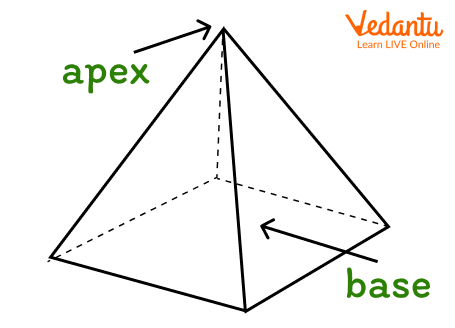But, the top is also considered as a base when it is parallel to the bottom.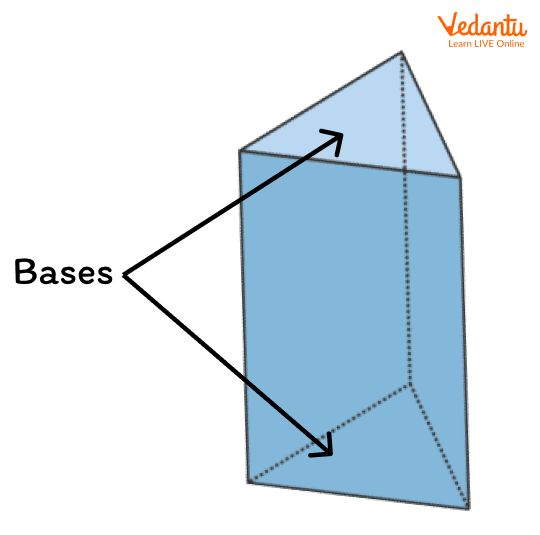### Base of Two-Dimensional Object

Let’s observe where the base is found in different 2-d shapes.

• The blue line in the following 2-D shapes represents the “Base” of the respective shapes.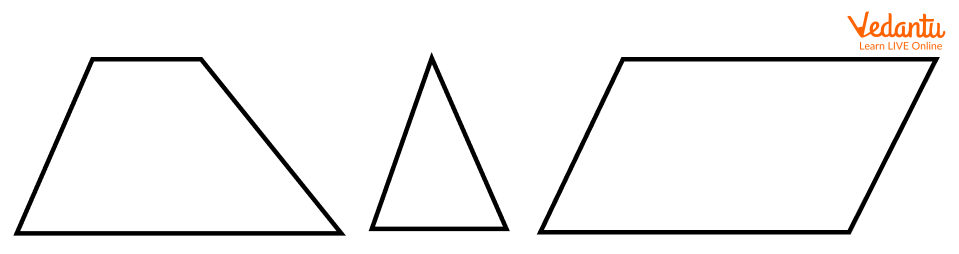The sides of 2-d shapes are also considered as base if the base and its corresponding height are perpendicular to each other. Look at the image below to understand precisely.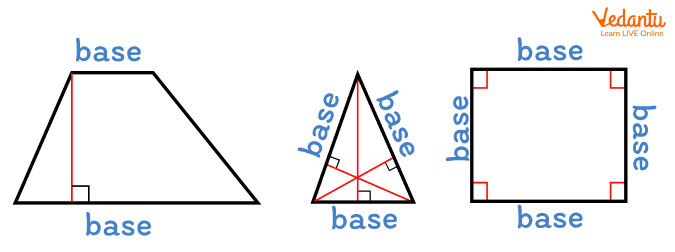Sometimes, the base of the shape needs to be extended to form its associated height.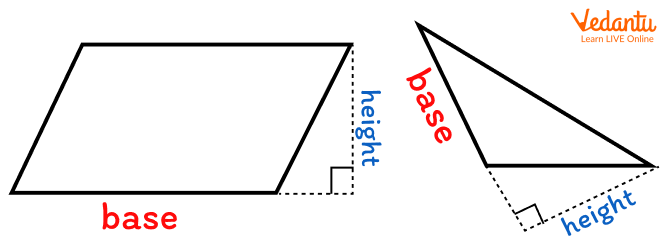### Base of Three-Dimensional Object

• The grey line in the following 2-D shapes represents the “Base” of the respective shapes.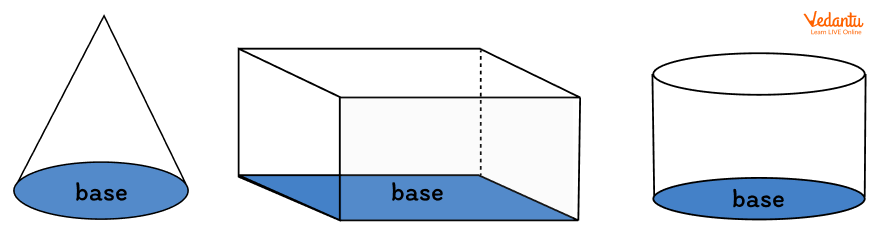Similar to 2-D shapes, other sides or faces are also considered as the base.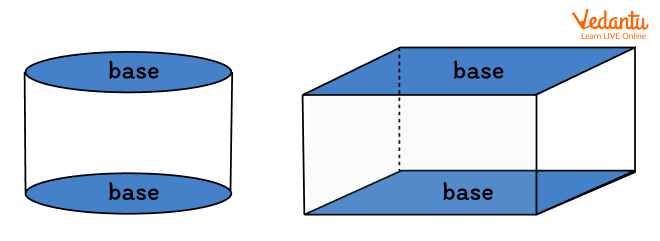## Base of a Shape Examples

• A triangle is a polygon with three sides. Any side of a triangle can be considered as its base while calculating its area. Once we choose the base of a triangle, there is only one line segment that represents its corresponding height.

• The base of a cone is the plane surface of a solid shape; the other face or side is a curved surface.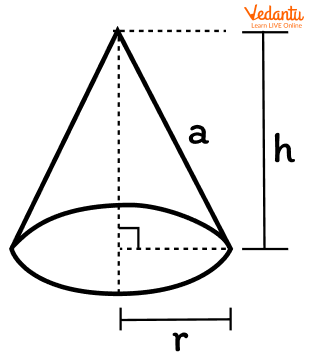• A trapezoid has two bases, one small base (b) at the top and one large base (B) at the bottom.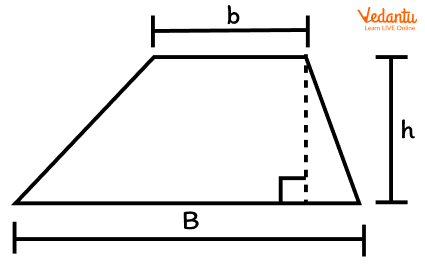## Conclusion

In short, the base is the surface upon which a solid object rests. A solid object may have one or more than one bases. For example, the prism has 2 bases. One base is at its top and the other at its bottom. Both are parallel to each other. Understanding the concept of the base is very important for kids as it is one of the fundamental concepts of Geometry. This will help to understand the complex geometrical concepts easily in higher classes.

Last updated date: 27th Sep 2023
Total views: 138.9k
Views today: 2.38k

## FAQs on The Base of a Shape: What is Base in Geometrical Shape?

1. Where are parallel bases found?

Parallel bases are found in a shape that has a pair of parallel sides. For example, the shape trapezoid has two bases with each base considered as one of the two parallel sides.

2. How can you identify the base of a shape?

The bottom part of any shape is considered as the base of a shape. For example, the bottom part of a triangle and rectangle is the surface or base of an object. The surface on which the solid object rests is also called the base.